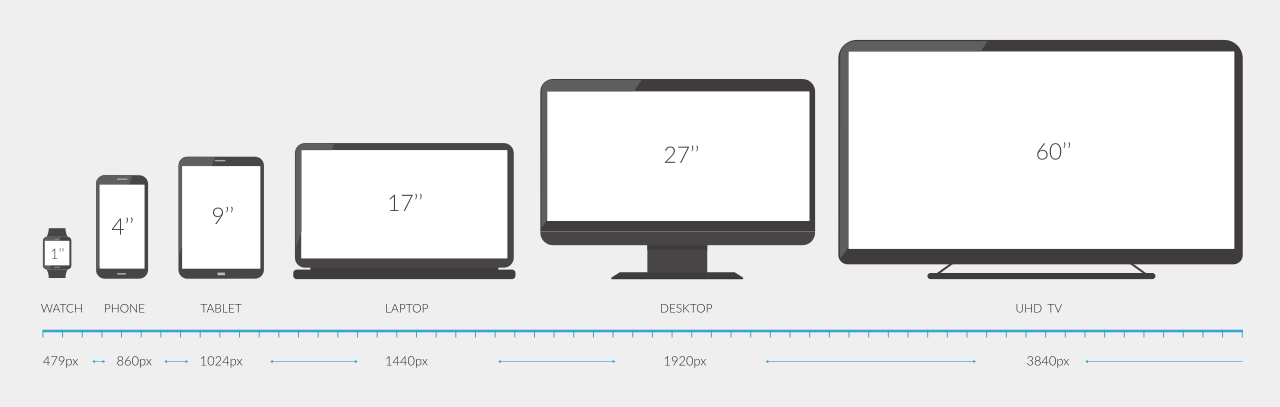# Screen Size Calculator

Calculate the total screen size given the aspect ratio and at least one dimension using the calculator below.

Enter One Screen Dimension

## Screen Size:

Learn how we calculated this below

## How to Calculate Screen Size

The size of a screen is the result of the diagonal measurement and the screen’s aspect ratio. The aspect ratio is the ratio of the screen’s width to its height.Whether you’re trying to figure out the dimensions of a TV or projector screen or figuring out the right computer monitor, the process for calculating screen size is the same.

There are three steps to calculating screen size; follow along as we go into detail on each one.

### Step One: Find the Aspect Ratio

The first step to calculating screen size is to find the screen’s aspect ratio. If you already know it, great, feel free to move on to the next step.

If you don’t already know the aspect ratio of the screen, try our aspect ratio calculator.

### Step Two: Find the Screen’s Diagonal

The next step to finding screen size is to find the screen’s diagonal measurement. This is the measurement in inches from one corner to the opposite corner of the screen.

In most cases, this is clearly marked for a TV or projector screen. If it isn’t, use a tape measure and measure from corner to corner to get the measurement.

### Step Three: Use the Screen Size Formula

Finally, use the screen size formulas to calculate the screen width and height dimensions.

Screen Width Formula:

screen width = ARw × diagonal / √(ARw2 + ARh2)

The screen width is equal to the aspect ratio width ARw times the screen diagonal divided by the square root of the aspect ratio width ARw squared plus the aspect ratio height ARh squared.

Screen Height Formula:

screen height = ARh × diagonal / √(ARw2 + ARh2)

The screen height is equal to the aspect ratio height ARh times the screen diagonal divided by the square root of the aspect ratio width ARw squared plus the aspect ratio height ARh squared.

The tool above uses these formulas and does the calculation automatically for you given any dimension.

## What is the Best Screen Size?

You might be wondering what the best screen size is? The answer is, it depends. The ideal screen size will vary depending on the application.

In some cases, a higher pixel density, or PPI, is the most important factor. So a smaller screen with a higher resolution might be better than a bigger screen.

Use our TV size and viewing distance calculator to find the right screen size for your application and resolution.